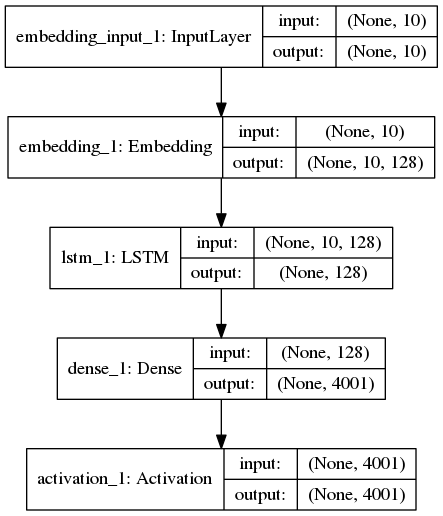#### 预处理

``````def load_data(self, path):
# read the entire text
text = open(path).read().strip().replace('\u3000', '').replace('\n', '')
print('corpus length:', len(text))

# all the vocabularies
vocab = sorted(list(set(text)))
print('total words:', len(vocab))

# create word-index dict
word_to_index = dict((c, i) for i, c in enumerate(vocab))
index_to_word = dict((i, c) for i, c in enumerate(vocab))

# cut the text into fixed size sequences
sentences = []
next_words = []
for i in range(0, len(text) - self.seq_length, self.step):
sentences.append(list(text[i: i+self.seq_length]))
next_words.append(text[i+self.seq_length])
print('nb sequences:', len(sentences))

# generate training samples
X = np.asarray([[word_to_index[w] for w in sent[:]] for sent in sentences])
y = np.zeros((len(sentences), len(vocab)))
for i, word in enumerate(next_words):
y[i, word_to_index[word]] = 1
``````

#### 定义模型

``````def load_model(self):
# load a Sequential model
model = Sequential()
model.add(LSTM(self.embed_size, input_shape=(self.seq_length, self.embed_size), return_sequences=False))
``````

#### 编译与训练

``````def compile_model(self, lr=0.01):
# compile the model
optimizer = RMSprop(lr=lr)
self.model.compile(loss='categorical_crossentropy', optimizer=optimizer)

def fit_model(self, batch_size=128, nb_epoch=1):
# fit the model with trainind data
self.model.fit(self.X, self.y, batch_size, nb_epoch)
``````

#### 生成

``````def _sample(self, preds, diversity=1.0):
# sample from te given prediction
preds = np.asarray(preds).astype('float64')
preds = np.log(preds) / diversity
exp_preds = np.exp(preds)
preds = exp_preds / np.sum(exp_preds)
probas = np.random.multinomial(1, preds, 1)

return np.argmax(probas)

def generate_text(self):
# generate text from randon text seed
start_index = random.randint(0, len(self.text) - self.seq_length - 1)

for diversity in [0.2, 0.5, 1.0, 1.2]:
print()
print('----- diversity:', diversity)

generated = ''
sentence = self.text[start_index: start_index + self.seq_length]
generated += sentence
print('----- Generating with seed: "' + sentence + '"')
sys.stdout.write(generated)

for i in range(400):
x = np.asarray([self.word_to_index[w] for w in sentence]).reshape([1, self.seq_length])
preds = self.predict(x)
next_index = self._sample(preds, diversity)
next_word = self.index_to_word[next_index]

generated += next_word
sentence = sentence[1:] + next_word

sys.stdout.write(next_word)
sys.stdout.flush()
print()
``````

``````Iteration: 2

----- diversity: 0.2
----- Generating with seed: "出战，不胜必斩！”庞"

----- diversity: 0.5
----- Generating with seed: "出战，不胜必斩！”庞"

----- diversity: 1.0
----- Generating with seed: "出战，不胜必斩！”庞"

----- diversity: 1.2
----- Generating with seed: "出战，不胜必斩！”庞"

``````